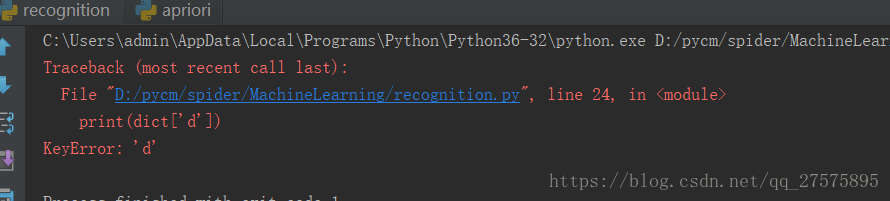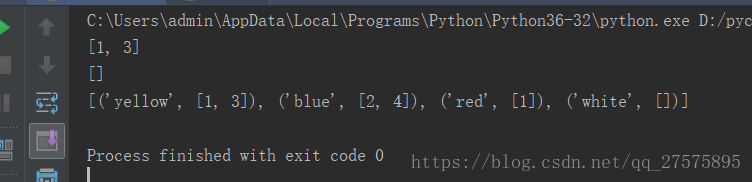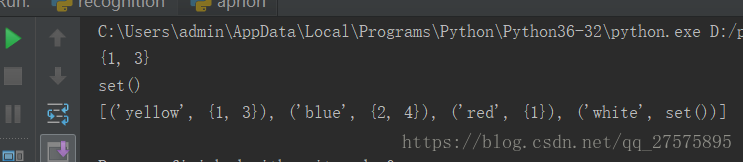# [Solved] django mysql\base.py error: KeyError:

### Tracking source code:

Problem file:`Python36\lib\site-packages\django\db\backends\mysql\base.py`

```    def get_new_connection(self, conn_params):
conn = Database. connect(** conn_params)
conn.encoders[SafeText] = conn.encoders[six.text_type]
conn.encoders[SafeBytes] = conn.encoders[bytes]
return conn```

### solution

1. Downgrade MySQLclient. Uninstall first, then install the specified version. `pip3 uninstall mysqlclient``pip3 install mysqlclient==1.3`.
2. My initial solution was to change the django code ( Python36\lib\site-packages\django\db\backends\mysql\base.py ), add an “if” as below:
```    def get_new_connection(self, conn_params):
conn = Database. connect(** conn_params)
conn.encoders[SafeText] = conn.encoders[six.text_type] #First
determine whether the bytes exist in the encoder, and if so, perform the operation
if bytes in conn.encoders:
conn.encoders[SafeBytes] = conn.encoders[bytes]
return conn```

# How to Fix keyerror in Python dictionary lookup

Python dictionaries usually look for keys directly, for example

``````dict={'a':1,'b':2,'c':3}
print(dict['a'])``````

So you want to look at print(dict[‘d’])
Since the dict doesn’t have the key in it, it’s just going to report an error, and python gives us a great way to do that, which is to use

``setdefault,The usage is as follows: dict.setdefault(key, [here set what you want the value to be if it doesn't exist, default is None]).``So here we can use this method to solve:

``print(dict.setdefault('d',0))``

If you want to add a new value to the dict, use setdefault. If you simply want to find the value, but the key does not exist, or you want to get a default value by reading the value through this key, then I recommend using defaultdict
I’ll start with this so-called Defaultdict from the Collections module, which is a collections module. Defaultdict (Function_factory) builds a Dictionary-like object where the value of the key is assigned by itself, but the type of value is an instance of a class in the Function_Factory and has a default value. Another concept introduced here is factory functions. Python’s factory functions are those built-in functions that are class objects, and when you call them, you’re actually creating an instance of a class. Int (), STR (),set(), etc.

``````import collections
s = [('yellow', 1), ('blue', 2), ('yellow', 3), ('blue', 4), ('red', 1)]
d = collections.defaultdict(list)
for k, v in s:
d[k].append(v)
print(d['yellow'])
print(d['white'])
print(list(d.items()))
``````

Our final output is as follows:We can see that when d has no corresponding key, it returns an empty list, because the factory function we used when we set defaultdict is list, and the default value of list is empty list. Now let’s see what would happen if the factory function was set()

``````import collections
s = [('yellow', 1), ('blue', 2), ('yellow', 3), ('blue', 4), ('red', 1)]
d = collections.defaultdict(set)
for k, v in s: